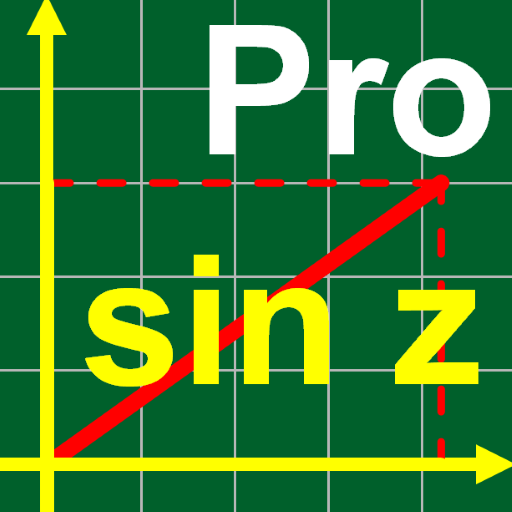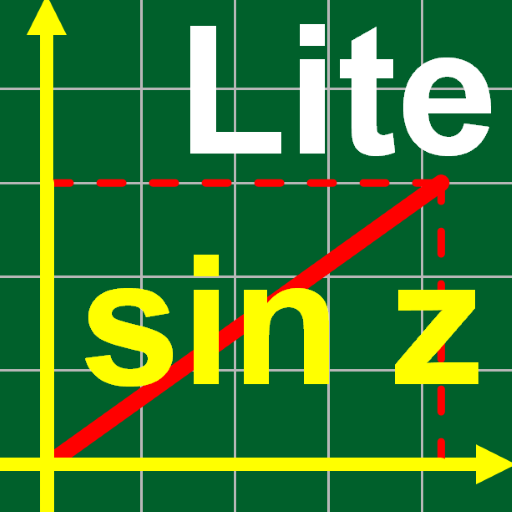# Complex Calculator

## Complex maths, including scientific functions

### Basic arithmetics and trigonometric, hyperbolic, exponential, logarithmic functions, etc.

Q = Question, A = Answer:
• Q1: Why does the app not use traditional algebraic input?
• A: Because RPN (reverse polish notation) is suitable ideally for handling of paired operators - such as complex numbers.
• Q2: What are the available display modes and their meaning?
• A: There are 4 display mode, which are cycled with the key "Disp" (plus optional digit).
• Fix n: Fix format, always n decimals behind the point
• Sci n: Scientific format, 1 digit before the point, n digits behind, plus exponent
• Eng n: Engineering format, similar to Sci, but the exponent is always a multiple of 3
• All: Shows always the available number of significant decimals
The first 3 formats above require the subsequent input of a single digit for the desired resolution. This is indicated in the small status line (above the numeric display) with the text "Enter n".
• Q3: Sometimes the calculation results don't fit inside the display, indicated by "..."?
• A: Reduce the number of displayed digits or turn the device to horizontal, which provides wider output fields.

• Q4: What has to be considered during operations in Hex and Oct mode?
• A: The calculation results are internally stored as double values. So there might be restrictions concerning accuracy and overflow, when dealing with very big figures.